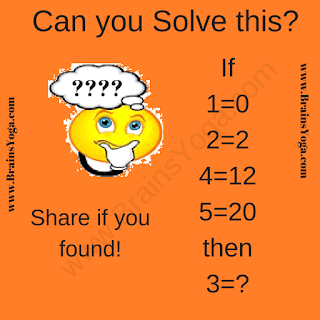Here is one hard logical reasoning puzzle which will twist your brain. In this logical reasoning puzzle, your challenge is to decode the logic of the equations and then solve the last equation to find the value of the missing number which will replace the question mark. So are you ready to crack this logical reasoning puzzle?Hard Logical Reasoning Puzzle for Teens

The answer to this "Hard Logical Reasoning Puzzle for Teens", can be viewed by clicking on the button. Please do give your best try before looking at the answer.

Fun With Puzzles said...

Answer is 6. If A=B is the given Mathematical equation then the logical expression which relates numbers in these equations is A*(A-1) = B

Unknown said...